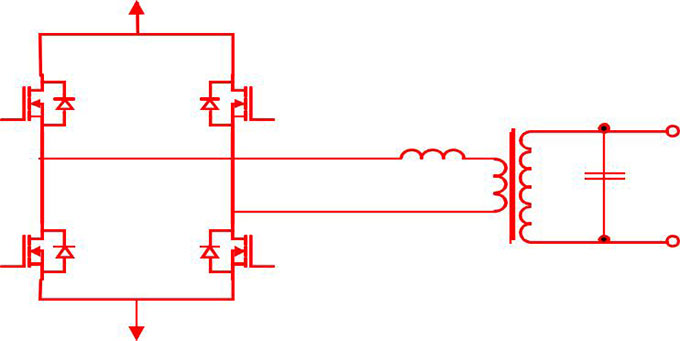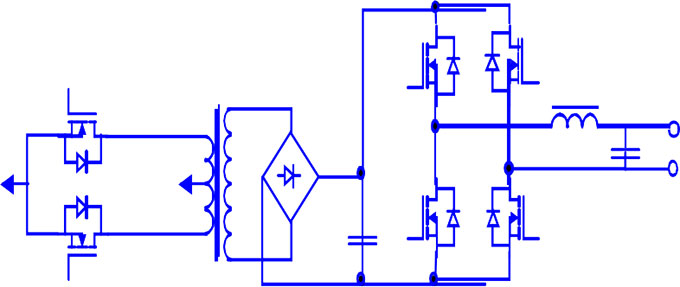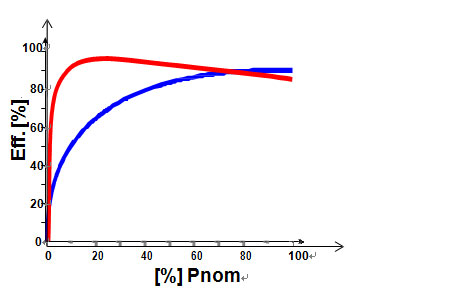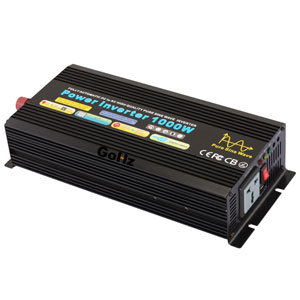Categories

# Power Inverter Basics

Basic knowledge of power inverter
Power inverter mainly adopts the PWM at present, controller switch to DC rapidly in a pulse-period to ensure that the DC integral value is equal to the sampling values of AC sine wave at the same time. After filtered, more than 96% sine wave output can be realized. Pulse inverter is self-excited inverter that output voltage is pulse modulation. This kind of power inverter reduces the voltage and electric current pulse number by increasing the cycle pulse switching times, to realize these only by increasing the commutation count of inverter.

The power inverter inductance of the AC determines the content of current harmonic. Therefore, in order to meet the requirements of grid access, you should guarantee the equivalent inductance value of photo-voltaic power generation system is small. Power inverter is connected to the low-pass filter and isolation transformer. It can filter out the harmonic below N-1, where N is trigger pulses flow of current period. To increase the switching frequency, the power loss of electronic devices will increase, but the loss of low pass filter will increase at low switching frequency. If you want to incorporate current clock multiplier of single-phase AC grid, the AC control signal frequency of Modulation PV generator DC output will double.

Basic Concept of power inverter
Usually, the AC electrical energy transforms into direct current (DC) can be called rectification, the circuit is known as rectifier circuit which completes the function of rectifier, the device achieve rectification process is called a rectifier device or rectifier equipment. Correspondingly, the process that the DC power transforms into AC power is called inverting, the circuit to complete inverter function known as the inverter circuit, the device realize the inverter process is called inverter device or power inverter.

Modern power inverter technology is a science to study inverter circuit theory and application. It is a practical technology based on industrial electronics, semiconductor technology, modern control technology, modern power electronic technology, semiconductor converter technology, pulse width modulation (PWM) technology and other subjects. It mainly includes the semiconductor power integrated device and its application, inverter circuit and the inverter control technology.

Classification of power inverter
There are many kinds of power inverters. They can be classified according to the different methods.

1. According to the inverter output AC power frequency, they can be divided into frequency inverters, intermediate frequency and high frequency inverters.

Frequency inverter
The frequency of power frequency inverter is 50/60Hz, figure 1 shows inverter circuit which uses a step-up transformer. It converts DC reverse into the frequency low-voltage AC power, then power frequency transformer booster into AC 220V, 50Hz to supply load. It has the advantage of simple structure, and various protective functions can be implemented at lower voltage. Between the inverter and the load have frequency transformer, so the inverter is stable, reliable operation and load capacity capability, impact resistant capability are strong, it also can restrain the higher harmonic wave component. However, the frequency transformer has bulky and expensive problem, and its efficiency is relatively low. The rated load efficiency of small power frequency inverter is not more than 90% at the current level. At the same time iron loss basically remain unchanged when the power frequency transformer running at full capacity and light load. So load loss is large and efficiency is low when running under light load.The inverter efficiency at low power output is more important than peak efficiency. Frequency inverter system is more suitable for isolated system (users load below the peak power at most of the time). Frequency inverter can sustain higher load power impact.

IF (intermediate frequency) inverter
Frequency of intermediate frequency inverter ranks 400Hz to 10000Hz.

High-frequency inverter
High-frequency inverter frequency is generally more than a dozen kHz to MHz. Figure 2 shows the power inverter circuit of high-frequency transformer. Low-voltage DC inverters for high-frequency low-voltage AC use high-frequency DC/DC converter technology. After a high-frequency step-up transformer, through the high-frequency rectifier circuit rectifies are usually the high voltage DC at 300V or more. Finally get 220V 50Hz frequency AC power for the load by frequency inverter circuits. Since the high-frequency inverter is used in small, lightweight high-frequency core material, thus the power density of circuit is greatly improved, so that the inverter no-load loss is small, the inverter efficiency is improved. In general, the peak energy conversion efficiency is over 90% for small and medium PVS high-frequency inverter.High frequency transformer topologyPower frequency transformer and the high frequency transformer efficiency curve, red for power frequency transformer, blue for high-frequency transformer

2. According to the number phases of the power inverter output, they can be divided into single-phase inverter, three-phase inverter and multiphase inverter.

3. According to the whereabouts of the inverter power output, they can be divided into active and passive inverter. Active inverter is the inverter output power to the industry grid, and passive inverter is the inverter output power to some electrical loads.

4. According to the form of inverter main circuit, they can be divided into single-ended inverter, push-pull inverter, half-bridge inverter and full-bridge inverter.

5. According to the power inverter main switching device types, they can be divided into thyristor inverters, transistor inverters, field effect inverters and insulated gate bipolar transistor (IGBT) inverters. It also can be summarized two categories as "semi-controlled type" inverter and "full control" inverter. The former do not have the self-turn-off capability, Component out of control after conduction, is called "semi-controlled" general thyristor. The latter has self-turn-off capability that is without the device turn-on and turn-off can be controlled by a control electrode, is called "full-controlled". The power field-effect transistors and insulated gate bipolar right transistor (IGBT) etc. fall into this category.

6. According to the DC power, they can be divided into voltage source inverter (VSI) and current source inverter (CSI). The former DC voltage nearly constant, the output voltage is the alternating square wave. The latter DC current nearly constant, the output current is also an alternating square wave.

7. According to the inverter output voltage or current waveform, they can be divided into non-sine wave inverter and pure sine wave inverter.

8. According to the power inverter control mode, they can be divided into Pulse Frequency Modulation (PFM) inverter and Pulse Width Modulation type (PWM) inverter.

9. According to the inverter switching circuit work mode, they can be divided into resonant inverter, fixed-frequency hard switching inverter and fixed-frequency soft switching inverter.

10. According to the inverter commutation mode, they can be divided into load converter inverter and self-commutation inverter.

Special feature power inverter
According to market demand, the inverter on the market in addition to having off-grid or grid functions also added many other features.

1. Off-grid backup inverter
(1) Static bypass inverter
At the battery or solar panel power supply shortage lead to sudden power loss, you can quickly cut into the mains power supply, switching time generally require less than 20ms (ensure that the server or PC does not power down, but resistive load such as light bulbs will flash phenomenon), you can set the priority switching when it works.
(2) Off-grid bidirectional inverter
In addition to static bypass inverter functions, it can also charge the battery through mains supply. It can ensure not lead to over-discharge when the battery voltage falls below a certain value. Some more powerful inverter can also be functions complemented between mains and inverter. Such as a rated capacity 7KW inverter need to give a total 10KW load, 7KW supply form inverter is not enough, and the general inverter 150% overload time no longer than a few minutes. In this case, the advantage of bi-directional inverter manifested, it may inverter 7KW, coupled with the mains supply 3Kw to supply 10KW load. This is very important when the system upgrade, if a normal system operation at rated power, in the case load slightly increase, either the system does not work, or you need to invest a lot of costs for system expansion, this is not worthwhile.

2. Grid connected back up inverter
Gird has a big drawback is that the output power with the light intensity changes. It can affect output power fluctuations for photovoltaic power plants, and it is a very dangerous thing for off-grid user near the gird. At this situation It is not allowed gird in some places, and then you have to use some of the energy storage device to power smooth. DC input voltage of grid-connected inverter is generally about several hundred volts, and the input of grid-connected inverter usually comes with MPPT. We all know that MPPT is not allowed to supply by constant current source, it can't be achieved that directly added to the battery in the input of the grid inverter. There is a solution that is to use a low voltage grid inverter to connect with a pure grid inverter output parallel. You can lap a power conversion device in the middle to ensure that the total output is constant. So that it can be supplied from the off-grid users.

Electronic energy-saving feedback load is actually a Power aging device, but also grid-connected inverter. But with a different grid-connected inverter is that its input voltage is constant, and allows a very low input voltage. With grid-connected backup inverter comparison, its output is generally constant output according to the setting.

Basics of power inverter selection
Technical performance
It has many basic parameters and technical conditions content to characterize power inverter performance, following I make a brief description only the evaluation of commonly used parameters.1. Rated output voltage
Within a predetermined fluctuation range of the DC voltage input allowed, which represents the rated voltage of the inverter can be output. The stability accuracy of the output voltage rating generally provides as follows:
(1) At steady-state operation, the voltage fluctuation range should be a limited, such as the deviation does not exceed the rating of ±3% or ±5%.
(2) At the mutation load (rated load 0%-50%-100%) or other interference factors affect the dynamic conditions which the output voltage deviation should not exceed the rating of ±8% or ±10%.

2. Output voltage imbalance
In the normal operating conditions, the three-phase unbalance voltage of inverter output (the ratio of negative sequence component and positive sequence component) should not exceed a specified value, usually expressed as a percentage, such as 5% or 8%.

3. Waveform distortion of the output voltage
It should provide permissible large waveform distortion (or harmonic content) when the inverter output voltage is sinusoidal. Generally it is represented by the total output voltage waveform distortion. Its value should not exceed 5% (single-phase output to 10%).

4. Rated output frequency
Inverter output AC voltage frequency should be a relatively stable value, usually 50Hz. Under normal operating conditions the deviation should be within ±1%.

Characterize the capacity of inverter inductive load or capacitive load. In the conditions of a sine wave, the load power factor is 0.7 to 0.9 (lag), rating is 0.9.

6. Rated output current (or rated output capacity)
It represents load power factor rated output current of the inverter within the specified range. The rated output capacity is given for some Inverter. The unit is VA or kVA. Inverter rated capacity is the product of rated output voltage and rated output current when the output power factor is 1 (i.e. purely resistive load).

7. Rated output efficiency
Inverter efficiency is the output power to input power ratio in the specified operating conditions, expressed as a percentage. Inverter efficiency is full load efficiency at rated output capacity, at 10% of rated output capacity efficiency is low load efficiency.

8. Protection
(1) Over-voltage protection: it should have output overvoltage protection measures for the inverter of no voltage stabilization measures to ensure the load doesn't be damaged by the output overvoltage
(2) Over-current protection: inverter over-current protection should be able to ensure that the load short-circuits current or exceeds the permitted value timely action to avoid damages from the surge currents.

9. Starting characteristics
It characterizes load starting performance and ability to dynamic work. The inverter should ensure reliable starting at rated load.

10. Noise
Transformers, filter inductors, magnetic switches, fan and other components of Power electronics equipment can produce the noise. When Inverter operates normally, the noise should not exceed 80dB, small inverter noise should not exceed 65dB.